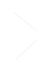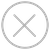TensorSpace.js
Getting StartBasic ConceptsTensorSpace ConverterModel PreprocessingModelsLayersMerge FunctionLayer Metrics
We can fetch the inputShape, outputShape and intermediate data from each layer instance.
Shape and Dimension
Suppose that there is a three-dimensional array x = np.array ([1,2], [3,4], [5,6], then its shape is x.shape = [2,2,2], which represents the length in each dimension. Its dimension x.shape.length = 3, indicating that the array makes sense in three dimensions.
filter_center_focus Dimension describes the dimensional characteristics of an array. For example, [1, 2, 3] is a one-dimensional array.
filter_center_focus Shapes describe the length characteristics of each dimension. For example, [[1, 2], [3, 4]] is a two-dimensional array of shapes [2, 2], representing a one-dimensional length of 2 and a two-dimensional length of 2.
filter_center_focus See Layer Dimension for more details.
Shape of inputs and outputs
The shape of some layers can change. The convolution layer, for example, adds a dimension and changes the value of length and width according to the characteristics of the convolution kernel (filter).
We provide the inputShape and outputShape properties to show the modifications from the inputs and the outputs of the layers.
In TensorSpace, the "dataFormat" is default to be "channel last". For example, shape = [28,28,3] is the input of a color image with 3 channels.
Intermediate layer data
In the neural network, data are transferred among different layers. After preprocessing, we can extract the required intermediate layer data and render them to the corresponding 3D visualizing objects. In this way, we can observe the data characteristics and changes of the previous data intuitively.
Code Snippet:
``````// add some layers
convLayer = new TSP.layers.Conv2d({...})